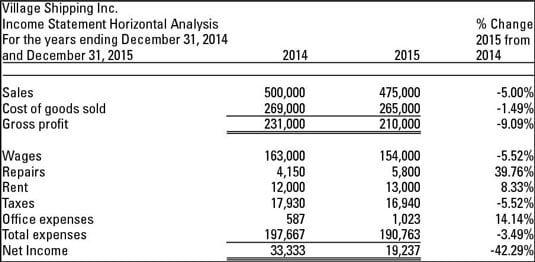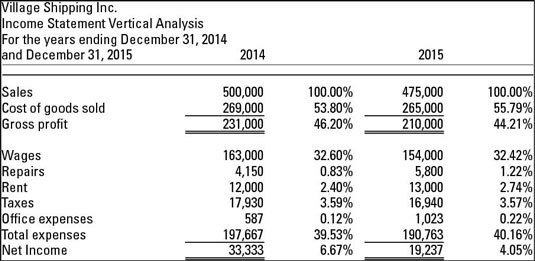A good way to do some ratio and trend analysis work is to prepare both horizontal and vertical analyses of the income statement. Both analyses involve comparing income statement accounts to each other in dollars and in percentages.

Understanding horizontal and vertical analysis is essential for managerial accounting, because these types of analyses are useful to internal users of the financial statements (such as company management), as well as to external users. If analysis reveals any unexpected differences in income statement accounts, management and accounting staff at the company should isolate the reasons and take action to fix the problem(s).

You can do the same types of analyses for balance sheet accounts. For a horizontal analysis, you compare like accounts to each other over periods of time — for example, accounts receivable (A/R) in 2014 to A/R in 2015.

To prepare a vertical analysis, you select an account of interest (comparable to total revenue) and express other balance sheet accounts as a percentage. For example, you may show merchandise inventory or accounts receivable as a percentage of total assets.

## Using horizontal analysis

Horizontal analysis compares account balances and ratios over different time periods. For example, you compare a company’s sales in 2014 to its sales in 2015.

The following figure is an example of how to prepare a horizontal analysis for two years. For useful trend analysis, you need to use more years (most investors use five), but this example gives you all the info you need to prepare a horizontal analysis for an unlimited number of years.The analysis computes the percentage change in each income statement account at the far right. The first number you might consider is the change in profit. Net income declined by 42.29 percent. Consider these two numbers and how they contributed to the decline:

• Repairs expense increased by 39.76 percent

• Sales declined by 5 percent

To isolate the reason for the net income decline, look at the change in total dollars, as well as the percentage change. The repair expense is the largest percentage change — an increase in costs. But note that the dollar amount of change is only \$1,650 (\$4,150 to \$5,800).

On the other hand, the sales decline was \$25,000 (\$500,000 to \$475,000). The decrease in sales has a bigger impact on the net income decline, when dollars are considered.

## Implementing vertical analysis

Vertical analysis restates each amount in the income statement as a percentage of sales. This analysis gives the company a heads up if cost of goods sold or any other expense appears to be too high when compared to sales. Reviewing these comparisons allows management and accounting staff at the company to isolate the reasons and take action to fix the problem(s).

The following figure is an example of how to prepare a vertical analysis for two years. As with the horizontal analysis, you need to use more years for any meaningful trend analysis. This figure compares the difference in accounts from 2014 to 2015, showing each account as a percentage of sales for each year listed.At the bottom of the analysis, note that net income, as a percentage of sales, declined by 2.62 percentage points (6.67 percent to 4.05 percent). As a dollar amount, net income declined by \$14,096 (\$33,333 to \$19,237). Management should consider both the percentage change and the dollar amount change.